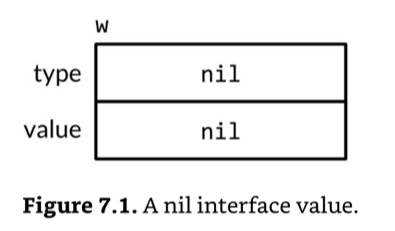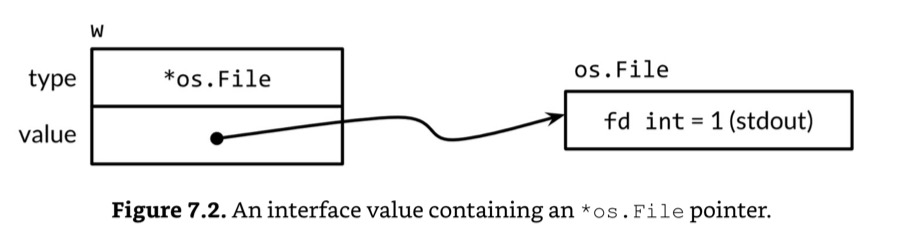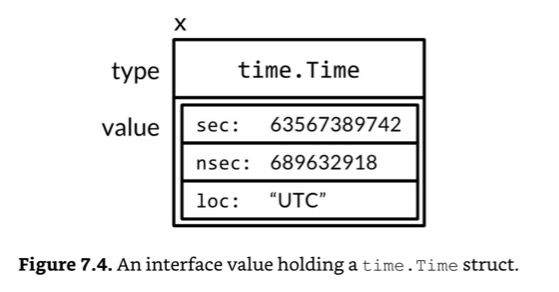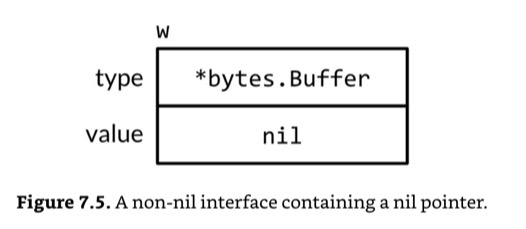# Basics of `interface`

``````package io

// Writer is the interface that wraps the basic Write method.
type Writer interface {
// Write writes len(p) bytes from p to the underlying data stream.
// It returns the number of bytes written from p (0 <= n <= len(p))
// and any error encountered that caused the write to stop early.
// Write must return a non-nil error if it returns n < len(p).
// Write must not modify the slice data, even temporarily.
//
// Implementations must not retain p.
Write(p []byte) (n int, err error)
}``````
``````package fmt

// The String method is used to print values passed
// as an operand to any format that accepts a string
// or to an unformatted printer such as Print.
type Stringer interface {
String() string
}``````
Combining interfaces like struct embedding
``````type ReadWriter interface {
Reader
Writer
}

type ReadWriteCloser interface {
Reader
Writer
Closer
}``````
• A type satisfies an `interface` if it possesses all the methods the interface requires.
The empty interface, `interface{}`, stands for any type.
``````var any interface{}
any = true
any = 12.34
any = "hello"
any = map[string]int{"one": 1}
any = new(bytes.Buffer)``````
Type assertions (`x.(T)`)
``````var w io.Writer
w = os.Stdout
f := w.(*os.File)      // success: f == os.Stdout
b := w.(*bytes.Buffer) // panic: interface holds *os.File, not *bytes.Buffer
// or, with `ok`
f, ok := w.(*os.File)      // success:  ok, f == os.Stdout
b, ok := w.(*bytes.Buffer) // failure: !ok, b == nil

// Testing would be like this
if str, ok := x.(Stringer); ok {}``````
Type Switches (`x.(type)`
``````switch x.(type) {
case nil:       // ...
case int, uint: // ...
case bool:      // ...
case string:    // ...
default:        // ...
}
// or, if you need to use the type asserted variable:
switch x := x.(type) {
case int:
fmt.Println(x) // `x` is now `int`
case int, uint:
// As there are type cases, the type of `x` is `interface{}` there.
fmt.Println(x)
}``````

# `interface` Values discussion

``var w io.Writer // nil interface value````w = os.Stdout````w = new(bytes,Buffer)````var x interface{} = time.Now()``An interface value assigned by `nil` of some type is not `nil`.
``````var b *bytes.Buffer // nil
var any interface{}

any = b

if any != nil {
fmt.Println("Gotcha!")
}``````
``````Gotcha!
``````• To prevent this subtlety, use `nil` interface, not the `nil` concrete type.